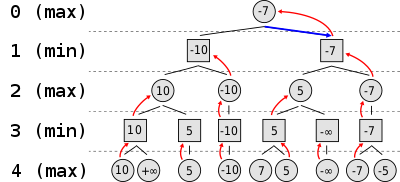# Minimax §

In Game Theory, minimax is a decision rule used to minimize the worst-case potential loss.

The value for a particular state is given by

•  is the index of the player of interest
•  denotes all other players except player
• is the action taken by player
•  denotes the actions taken by all other players
• is the value function of player

This value function for player is calculated in a worst-case approach: player considers the best opponent responses to his strategies (who tries to minimize), and selects the strategy such that the opponent’s best strategy gives a payoff as large as possible (i.e. maximize).

Serendipity I also see this in F1TENTH. This is the most common framework in adversarial settings. ohh so CFR is the minimax version for Imperfect Information games!

##### A side note on “Expectiminimax” §

Actually, you can have chance events and still be Perfect Information, such as Backgammon. You run the expectiminimax algorithm, which simply takes the expected value of the chance nodes, see walkthrough example here.

### Representation + Implementation §

Minimax represents winning conditions as (-1) for one side and (+1) for the other side. We implement a minimax algorithm by doing Depth-First Search.

To explain this to people, you sort of need to reason backwards with them.

Good article: https://philippmuens.com/minimax-and-mcts

Also, when we refer to minimax, we often refer to the Depth-Limited version of minimax, since most games are already too big to traverse the entire game tree.#### Pseudocode §

This is for the Depth-Limited Search version.

py
def minimax(state, max_depth, is_player_minimizer):
if max_depth == 0 or state.is_end_state():
# We're at the end. Time to evaluate the state we're in
return evaluation_function(state)

# Is the current player the minimizer?
if is_player_minimizer:
value = -math.inf
for move in state.possible_moves():
evaluation = minimax(move, max_depth - 1, False)
min = min(value, evaluation)
return value

# Or the maximizer?
value = math.inf
for move in state.possible_moves():
evaluation = minimax(move, max_depth - 1, True)
max = max(value, evaluation)
return value`

The minimax function returns a heuristic value for leaf nodes (terminal nodes and nodes at the maximum search depth).

### Random §

In two-player zero-sum games, the minimax solution is the same as the Nash Equilibrium.

Ahh this explains: “In perfect-information games, the value that is substituted at a leaf node of a depth-limited subgame is simply an estimate of the state’s value when all players play an equilibrium”.

### Next §

Depth-Limited Minimax is still not enough. We can optimize even more. See Alpha-Beta Pruning.